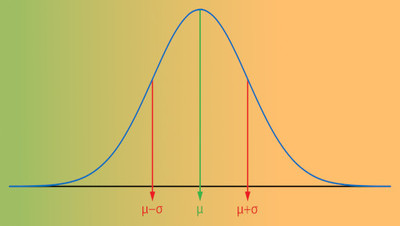# Statistics I

used at Portland Community College

Educator Edition

 Course MTH 243, Statistics I. Introduces displaying data with graphs, numerical descriptions of data, producing data, elementary probability, probability distributions, confidence intervals and significance testing. Investigates applications from science, business, and social science perspectives. Graphing calculator with advanced statistical programs and/or computer software required; see instructor. Textbook This course is frequently paired with the following textbook(s): Stats Data and Models by DeVaux, Velleman, and Bock Prerequisites Intended for students who have passed an Intermediate Algebra course.

## Customize your Student Experience

Edfinity is a full-featured homework system. Use this problem series as-is, or mix-and-match with other series or your own problems as desired. Edfinity gives you complete control over the every aspect of students' online assessment experience.

## Intended Outcomes

Upon completion of the course students should be able to:

1. Analyze data and graphs in real world scenarios to recognize what probability and statistics are appropriate, formulate problems about the scenarios, creatively model these scenarios (using technology, if appropriate) in order to solve the problems using multiple approaches, then judge if the results are reasonable and clearly interpret the results via written or oral communication.
2. Recognize probability and statistics concepts that are encountered in the real world, understand and be able to communicate the underlying mathematics involved to help another person gain insight into the situation.
3. Work with probability and statistics in various situations and use correct mathematical terminology, notation, and symbolic processes in order to be prepared for future coursework and to continue a course of study in their major field that requires the use of and an understanding of the concepts of probability and statistics.
4. Read a peer reviewed journal article and understand the meaning and logical implications behind the statistical processes of a confidence interval and a significance test.

## Powered by WeBWorK

This problem series includes WeBWorK-enhanced problems. WeBWorK problems are highly sophisticated, supporting the representation and manipulation of mathematical objects such as vectors, points, matrices, intervals, and complex numbers, with customizable answer checkers. Students are encouraged to make multiple attempts until they succeed, and given immediate feedback on correctness.

Edfinity provides full access to the WeBWorK Open Public Library (OPL), a collection of over 20,000 rich, interactive problems across a wide range of math and science subjects, including college algebra, discrete mathematics, probability and statistics, single and multivariable calculus, differential equations, linear algebra and complex analysis.

## Problem Sets

1. Edfinity Demo
2. Basics Of Data
3. One Variable Calculations
4. Displaying Data
5. Z Score Basics
6. Normal Distributions
7. Empirical Rule
8. Normal Area For Arbitrary Z
9. Sampling Issues
10. Experimental Design
11. Basic Probability
12. General Probability
13. Venn Diagrams And Sample Spaces
14. Conditional Probability
15. Expected Value And Variance
16. Binomial Distribution
17. Distribution Of Sample Mean
18. Sampling And Central Limit Theorem
19. Proportion And Confidence Intervals
20. Significance Testing
21. Scatterplots And Linear Regression

##License

This is a multi-student, educator license. You will be able to manage an online course with your students, and monitor their progress.

Price: FREE
Trusted by## Recommendations

Cookies help us deliver our services. By using our services, you agree to our use of cookies.